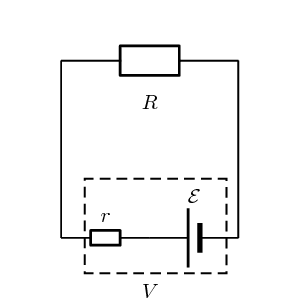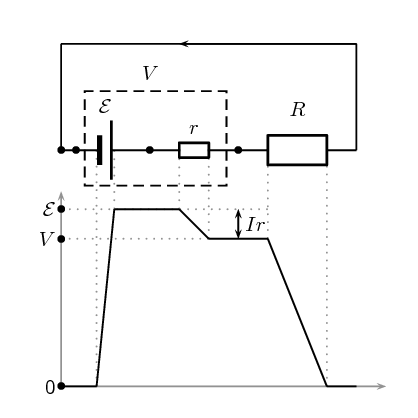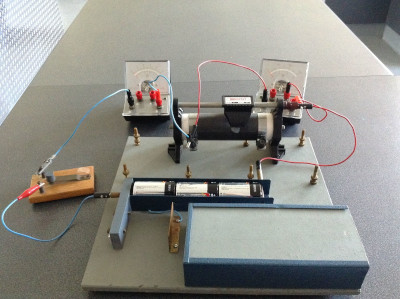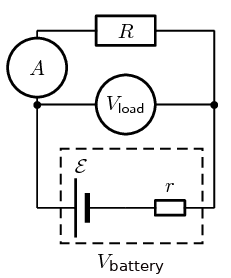Home Practice
For learners and parents For teachers and schools
Textbooks
Full catalogue
Pricing SupportLog in

We think you are located in United States. Is this correct?

# 10.3 Batteries and internal resistance

## 10.3 Batteries and internal resistance (ESCPV)

Up until now we have been dealing with ideal batteries in that they aren't affected by the circuit or current in any way and provide a precise voltage until they go flat.

If you measure the potential difference across the terminals of a battery on its own you will get a different value to what you measure when it is in a complete circuit. The value will be less when the battery is included in a complete circuit. Sometimes the difference is called the lost volts. Nothing has actually been lost but energy has been transferred.

Real batteries are made from materials which have resistance. This means that real batteries are not just sources of potential difference (voltage), but they also possess internal resistance. If the total potential difference source is referred to as the emf, $$\mathcal{E}$$, then a real battery can be represented as an emf connected in series with a resistor $$r$$. The internal resistance of the battery is represented by the symbol $$r$$.The external resistance in the circuit is referred to as the load.

Suppose that the battery with emf $$\mathcal{E}$$ and internal resistance $$r$$ supplies a current $$I$$ through an external load resistor $$R$$. Then the potential difference across the load resistor is that supplied by the battery:

$V_{\text{load}}=I\cdot R$

Similarly, from Ohm's Law, the potential difference across the internal resistance is:

${V}_{\text{internal resistance}}=I\cdot r$

The potential difference $$V$$ of the battery is related to its emf $$\mathcal{E}$$ and internal resistance $$r$$ by:

\begin{align*} \mathcal{E}& = V+Ir\\ &\text{or}\\ V& = \mathcal{E}-Ir \end{align*}

The battery is the source of energy and the energy provided per unit charge (emf) passing through the battery is equal to the total work done (potential difference) across the components in the circuit. This can be illustrated by showing the energy per unit charge as as function of the position in the circuit. The charge gains energy when moving through the battery and loses energy when moving through resistors.

1. Postive work is done on a unit charge by the battery transferring an energy equal to the emf.
2. The charge does work to overcome the internal resistance of the battery. Doing work requires that the charge lose some energy. The work done to overcome the internal resistance is $$V_{\text{internal resistance}}=Ir$$.
3. When the unit charge leaves the battery it has less energy than the original emf. This is now the total energy that it can use to do work moving through the circuit, $$V_{\text{load}} = \mathcal{E}-Ir$$.
4. As the charge moves around the circuit it does work passing through each component which is equal to $$IR$$.The emf of a battery is essentially constant because it only depends on the chemical reaction (that converts chemical energy into electrical energy) going on inside the battery. Therefore, we can see that the potential difference across the terminals of the battery is dependent on the current drawn by the load. The higher the current, the lower the potential difference across the terminals, because the emf is constant. For the same reason, the potential difference only equals the emf when the current is very small.

The current that can be drawn from a battery is limited by a critical, maximum value $${I}_{c}$$. The more resistance in the circuit the less the current will be. Imagine that you have a wire with no resistance that you use to connect the terminals of the battery. The circuit is complete, current will flow and adding any resistance to the circuit would decrease the current. The current without any external resistance will be $${I}_{c}$$. At a current of $${I}_{c}$$, $$V=\text{0}\text{ V}$$ because there is no load in the circuit. Then, the equation becomes:

\begin{align*} \text{0}& = \mathcal{E}-{I}_{c}r\\ {I}_{c}r& = \mathcal{E}\\ {I}_{c}& = \frac{\mathcal{E}}{r} \end{align*}

The maximum current that can be drawn from a battery is less than $$\frac{\mathcal{E}}{r}$$.

## Worked example 6: Internal resistance

Determine the internal resistance of a battery that has an emf of $$\text{12,00}$$ $$\text{V}$$ and has a potential difference across its terminals of $$\text{10,00}$$ $$\text{V}$$ when a current of $$\text{4,00}$$ $$\text{A}$$ is flowing through the battery when connected in a circuit.

### Determine how to approach the problem

It is an internal resistance problem. So we use the equation:

\begin{align*} \mathcal{E}& = V+Ir \end{align*}

### Solve the problem

\begin{align*} \mathcal{E}& = V+Ir\\ \text{12}& = \text{10}+\text{4}\left(r\right)\\ r & = \text{0,5}\text{ Ω} \end{align*}

The internal resistance of the battery is $$\text{0,50}$$ $$\text{Ω}$$.

temp text

## Finding the internal resistance of a battery

### Aim

To determine the internal resistance of a battery.

### Apparatus

You will need the following items for this experiment:

• battery / cell to be studied

• a set of resistors or a rheostat

• ammeter

• voltmeter

### Method

We will set up a circuit that contains the battery we want to study in series with a resistor. Then we will measure the potential difference across the load as well as the current for a number of different resistors/rheostat in the circuit. It doesn't matter if it is a different resistor each time or more resistors in series or parallel. What matters is that the overall resistance of the circuit changes so that the current is different each time. The reason that doing this can tell us about the internal resistance of the battery is because the potential difference across the internal resistance is $$V_{\text{internal resistance}}=I\cdot r$$ and we can vary I by changing the resistance of the circuit. If the potential difference across the internal resistance is changing and we add up all the potential differences, $$\mathcal{E} = V_{\text{load}}+V_{\text{internal resistance}}$$ we can determine the internal resistance.To do this we will actually plot a graph of $$V_{\text{load}}$$ versus $$I$$ and then use the features of the graph to determine $$\mathcal{E}$$ and $$r$$. To understand why plotting the graph will help us we start with the equation for the magnitude of the $$\mathcal{E}$$ and substitute Ohm's Law and re-arrange as follows: \begin{align*} \mathcal{E} & = V_{\text{load}} + V_{\text{internal resistance}} \\ \mathcal{E} & = V_{\text{load}} + I\cdot r \\ V_{\text{load}}& = \mathcal{E} -I\cdot r \\ V_{\text{load}}& = -r\cdot I + \mathcal{E} \\ \underbrace{V_{\text{load}}}_{y}& = \underbrace{-r}_{m}\cdot\underbrace{I}_x + \underbrace{\mathcal{E}}_{c} \end{align*} If we plot $$V_{\text{load}}$$ versus $$I$$ we will be plotting data that are governed by this relationship. This allows us to conclude that the slope of the graph, $$m$$, will be $$-r$$ and the intercept vertical axis, $$c$$, will be the emf $$\mathcal{E}$$.

### Results

Record your results in a table like the one below. You can take more readings if you like.

 Setup $$V_{\text{load}}$$ ($$\text{V}$$) $$I$$ ($$\text{A}$$) Resistance 1 Resistance 2 Resistance 3 Resistance 4 Resistance 5

### Discussion and conclusion

Plot your data on a set of axes similar to this example. The blue crosses represent the measured data points, the gray, dashed line is the drawn straight line through the data points. The best fit line you draw doesn't need to go through all the data points, it should, in general, have as many points above and below the line. The slope of the line can be measured and equated to $$-r$$ and the intercept with the vertical axis will give you $$\mathcal{E}$$. The intercept with the horizontal axis would give you the maximum possible current the battery could deliver.

• Do your data form a perfectly straight line?
• What errors were introduced?